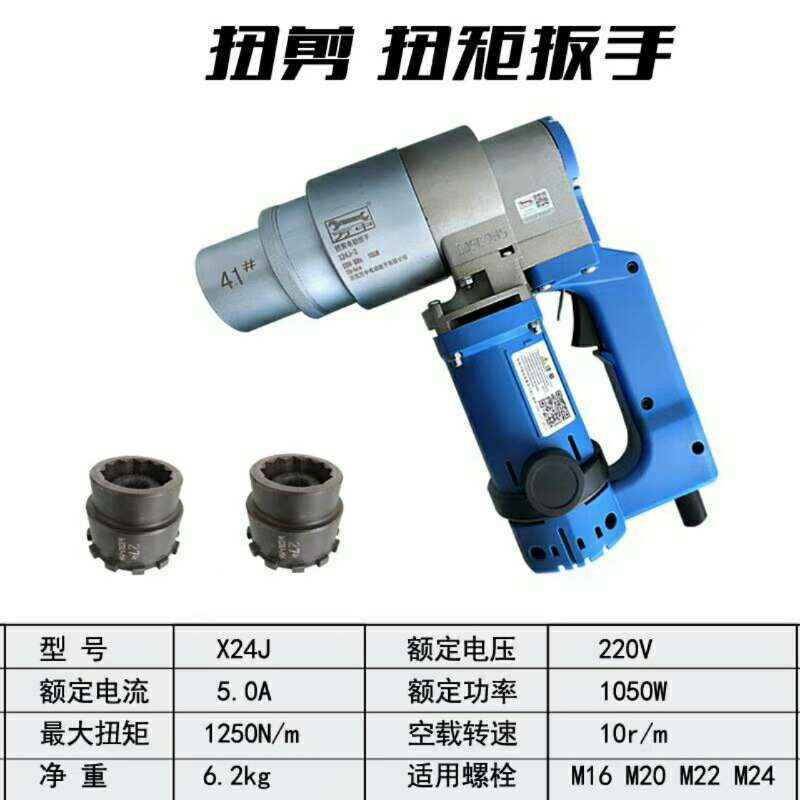13406879330

# 高强螺栓等级标准

The degree of strong Bai is divided into Du grades according to the mechanical properties. According to relevant standards, the performance grade of bolts can be divided into more than 10 grades, such as 3.6, dao4.6, 4.8, 5.6, 6.8, 8.8, 9.8, 10.9 and 12.9. Among them, bolts of grade 8.8 and above are made of low-carbon alloy steel or medium carbon steel and heat-treated (quenching and tempering), which are generally called high-strength bolts, and the rest are commonly referred to as ordinary bolts. The bolt performance grade label consists of two parts, which respectively represent the nominal tensile strength and yield strength ratio of bolt material. For example:

The meaning of bolts with performance grade 4.6 is as follows:
1、螺栓材质公称抗拉强度达400MPa级；
1. The nominal tensile strength of bolt material is 400MPa;
2、螺栓材质的屈强比值为0.6；
2. The yield strength ratio of bolt material is 0.6;
3、螺栓材质的公称屈服强度达400×0.6=240MPa级3. The nominal yield strength of bolt material is 400 × 0.6 = 240mpa

After heat treatment, the material of high strength bolt with performance grade of 10.9 can reach the following requirements:
1、螺栓材质公称抗拉强度达1000MPa级；
1. The nominal tensile strength of bolt material reaches 1000MPa;
2、螺栓材质的屈强比值为0.9；
2. The yield strength ratio of bolt material is 0.9;
3、螺栓材质的公称屈服强度达1000×0.9=900MPa级
3. The nominal yield strength of bolt material is 1000 × 0.9 = 900MPa